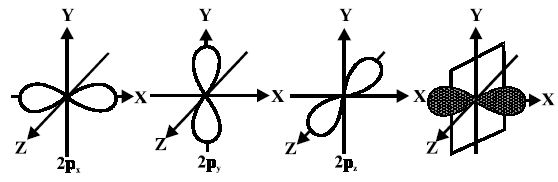Chemistry

# Explain Shape of P-Orbitals

For p-orbitals or p-subshell, Ɩ = 1, there are three values of m namely -1, 0, +1. It means that p orbitals can have three possible orientations. These three p-orbitals are equal in energy (degenerate state) but differ in their orientations. Each p-orbital consists of two lobes symmetrical about a particular axis. Depending upon the orientation of the lobes, these are denoted as 2px , 2py and 2pz accordingly as they are symmetrical about X, Y and Z – axis respectively.The lines in the figure represents the cross-section of the three-dimensional boundary surface of p-orbitals. The boundary surface means the surface which encloses 90 percent of the dots representing the electrons. Two lobes of each porbital are separated by a nodal plane (a plane having zero electron density). For example, for 2px orbital, YZ plane is the nodal plane x.

Thus, p-orbitals have dumb-bell shape and have directional character. The probability of finding the electron is equal in both the lobes. The p-orbitals of higher energy levels have similar shapes although their size is bigger.